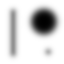#Gaussian blur demo

## Description

This program loads a picture into memory, computes a Gaussian blur on it according to the radius selected by the user, and draws the resulting blurred image. This demonstrates how my open-source fast Fourier transform (FFT) library can be used in a practical application (image convolution) with acceptable runtime performance in JavaScript. The source code is available for viewing. Filtering an image with Gaussian blur is a common feature available in image editor applications, such as Adobe Photoshop and GIMP.

## Notes

• In my implementation here (and not necessarily speaking for other implementations), the blur radius is defined as the standard deviation of the Gaussian distribution. The distribution has no true “radius” because it has an exponentially decreasing tail that never reaches zero.

• The Gaussian blur implemented here is performed in the linear domain, assuming an image and display gamma of 2.2 (not sRGB out of laziness). Thus the input image is converted from the gamma domain to the linear domain, Gaussian-blurred, and converted back to the gamma domain. This gives better color and brightness accuracy than a naive convolution performed directly over the gamma domain without linearization.

• Like any convolution on finite data, the Gaussian blur will attempt to get data beyond the edges of the image, but that data is unknown by definition. There are a number of possible ways to handle this (such as replicating edge pixels), but my chosen way is to add an extra channel as a weight reference. The weight channel is a white rectangle with the same dimensions as the image, surrounded by an infinite border of black. The weight channel is blurred with the same kernel as the RGB channels. The final RGB image is equal to the blurred RGB image divided by the blurred weight channel. (As an implementation detail, the weight channel makes it possible to not normalize the kernel or the convolutions; a single normalization is performed at the very end.)

• The JavaScript code contains a number of algorithmic optimizations to make it less painful as a real-time demo. The optimizations implemented include pre-computing some of the input image conversions, cutting off the convolution kernel when the coefficients get too small, choosing the smallest FFT length that fits the kernel and image, caching FFT tables and the transformed kernel, and doing two real convolutions for the cost of one complex convolution by using the real and imaginary components. On a desktop computer with an Intel Haswell CPU, it takes between 150 to 300 ms to blur a 600×400 image, depending on the blur radius. Furthermore, I tried hand-writing the core FFT and convolution code in the language subset called asm.js, but it produced no speedup in my Mozilla Firefox 49 compared to simply writing clear JavaScript code and letting the advanced tracing JIT compiler do its job.

• A Java implementation of Gaussian blur is provided here: GaussianBlurDemo.java. Usage: `java GaussianBlurDemo Input.png/bmp Radius Output.png`. Because it is targeted for batch processing, it forgoes the speed optimizations present in the JavaScript code, and achieves simpler logic and subtly higher image quality. For example, the Java code doesn’t truncate the Gaussian kernel for simplicity, it uses double-precision floating point instead of single precision, and doesn’t convolve two channels simultaneously (so there is no FFT crosstalk). When comparing just the core of the computation, which is the part of the JavaScript code that executes every time the radius is changed (so skipping the input image conversion precomputation, but including the kernel computation, convolutions, and image output conversion), the Java version is about 4× faster than the JavaScript version on my machine.

• The demo image was photographed and edited by Project Nayuki.